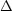# Microwave background radiation - temperature at recombination

• I
Gold Member
TL;DR Summary
Is there an upper limit for the temperature of recombination (last scattering)?
The commonly called value of the temperature at recombination is 3000 K.

According to this reference the process of recombination can be described by the Saha equation:

3.1. Recombination and the formation of the CMB
Recombination happens quickly (i.e., in much less than a Hubble time t ~ H-1), but is not instantaneous. The universe goes from a completely ionized state to a neutral state over a range of redshiftsz ~ 200. If we define recombination as an ionization fraction Xe = 0.1, we have that the temperature at recombination TR = 0.3 eV.

Whereby 0.3 eV equals 3480 K.​

Another reference:

It is mainly determined by the ionization potential of hydrogen and the baryon-to-photon ratio. ne ~ 500 cm-3 (roughly same as Galactic HII regions) Te = Tr = 2970 K = 0.26 eV

Tr = 3000 K fits well to the redshift z ~ 1100 of the CMB (according to the L-CDM model) with respect to the 2.725 K we measure it today. I wonder if there is an upper limit for Tr.

The cosmological model of Alexandre Deur claims Such fit yields H0 = 72.99 ± 0.06 km/s/Mpc, zL = 1728 ± 1

Redshift z = 1728 of the CMB would suggest that Tr is around 4700 K however. Considering plasma physics would you say that's still reasonable?

Last edited:
•vanhees71 and ohwilleke

Gold Member
2022 Award
••pinball1970, Bandersnatch, Jorrie and 1 other person
Gold Member
Thank you very much for this very informative and trustworthy reference.

So the conclusion is:

Using equilibrium theory, which is an oversimplification, gives the following fractions of neutral hydrogen for three different temperatures

• At T=3000K the Universe would have been more than 99% neutral
• At T=4000K the Universe would have been about 40% neutral
• At T=3800K the Universe would have been about 70% neutral
Using a more correct 3-level model developed by Peebles and, independently, by Zel’dovich, gives that the Universe would have been about 90% neutral by the time the temperature had dropped to T=3000K. It is this temperature which is usually quoted when we talk about the temperature of the Universe when recombination (decoupling) occurred.

This matches very well the observed redshift z = 1100.

Last edited:
•vanhees71
Hyperfine
The following paper may be of interest: Sara Seager, Dimitar D. Sasselov, and Douglas Scott, "How Exactly Did the Universe Become Neutral?" Astrophys.J.Suppl. 128 407-430 (2000).

Abstract said:
We present a refined treatment of H, He I, and He II recombination in the early Universe. The difference from previous calculations is that we use multi-level atoms and evolve the population of each level with redshift by including all bound-bound and bound-free transitions. ...

https://arxiv.org/abs/astro-ph/9912182